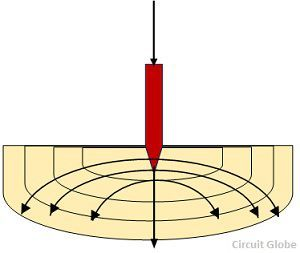# Earth Resistance

Definition: The resistance offered by the earth electrode to the flow of current into the ground is known as the earth resistance or resistance to earth. The earth resistance mainly implies the resistance between the electrode and the point of zero potential. Numerically, it is equal to the ratio of the potential of the earth electrode to the current dissipated by it. The resistance between the earthing plate and the ground is measured by the potential fall method.

The resistance of the earthing electrode is not concentrated at one point, but it is distributed over the soil around the electrode. Mathematically, the earth resistance is given as the ratio of the voltage and the current shown below.Where V is a measured voltage between the voltage spike and I is the injected current during the earth resistance measurement through the electrode.

The value of the earth resistance for different power stations is shown below

Large Power Station – 0.5 ohms
Major Power Station – 1.0 ohms
Small Substation – 2.0 ohms
In all other cases – 8.0 ohms

The region around the earth in which the electrode is driven is known as the resistance area or potential area of the ground. The fault current which is injected from the earth electrode is passing away from the electrode in all directions shown below in the figure. The flow of current into the grounds depends on the resistivity of the soil in which the earth electrode is placed. The resistivity of the soil may vary from 1 to 1000 ohm-m depends on the nature of the soil.The resistivity of the earth depends on its temperature. When the temperature is greater than 0ºC, then its effect on ground resistivity is negligible, But at 0ºC the water in the soil starts freezing which increase their resistivity. The resistivity of the earth is also affected by the composition of some soluble salts as shown in the figure below.The resistance of the earth varies from layer to layer. The lower layer of soil has more moisture and lower resistivity. If the lower layer contains hard and rocky soil, then their resistivity increases with depth.